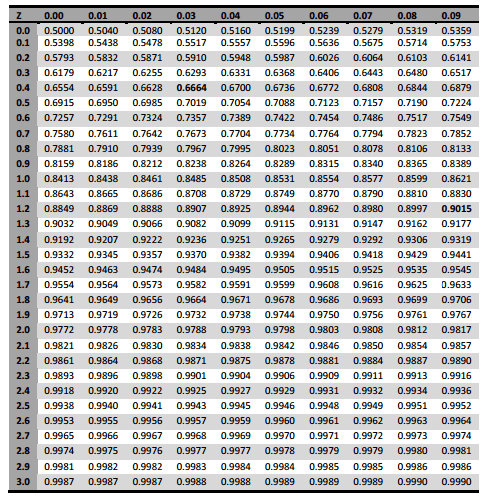# Statistics problem using cumulative from mean

MarcL
Sorry if this is in the wrong section. I didn't know which homework section I should use.

1. Homework Statement

Human subjects are involved in a medical study to represent certain segments of the population in terms of blood lead concentration, PbB. First, assume the normal distribution properly represents the population of children with the mean concentration of 22.5 μg/dl and the standard deviation σ of 5.8 μg/dl. Then, determine the range of PbB values (minimum and maximum) that should be used for the top 3 % of PbB values in the population. Use the cumulative density function table provided.

## Homework Equations

here's the table:Also z = (X - μ) / σ where μ is the mean and σ is the standrad deviation.

## The Attempt at a Solution

I'm not too sure on what I did... might be completely off. I used the following logic. If it is the top 3%, then we can find the maximum density using 0% as our total. Therefore, we would need to have Z = 3.9 on both side of the probability ( by that I mean P( 3.9 < Z < 3.9) ). So using that idea, we can find Xmax using the following:

3.9 = (X - 22.5) /5.8 --> 45.15 ug/dl

For the the Xmin , also the lost integer in the interval, I used the following:
φ(z=3.9) - φ(z= y ) = .03 --> .9999 - y = .03 --> y=.9699
Therefore, using the table .9699 will give you 1.88.
Using the same method as above, 1.88 will give x as 33.40 ug/dl

This whole thing seems completely wrong though. I've never taken a stat class before and this was handed to me. Using the best of my abilities ( and wikipedia) to try to understand.

Homework Helper
I think you did the right method for the top 3%. There is no need to find a max, since normal distribution technically goes to infinity. You are looking for the Z value associated with 97%, thus leaving 3% above that number (.9699 is as close as you need to get). From a mathematical standpoint, you would have a range from 33.4 to infinity. If you need an upper bound, I don't see why anyone number would be better than the one you used.

lp560-4
for you Xmax, you're z value should be 3.09 not 3.9. Goodluck on the engr202 assignment

masterblaster
lp, why should it be 3.09?

Funny how we are all in the same class

lp560-4
well to be honest I only understood how to do it thanks to his example but if you look at the cell containing 0.9990, its in row 3.0 and column 0.09, so z is 3.09
same goes for the Xmin. cell 0.9699 is in row 1.8 and column 0.08, so z = 1.88

Homework Helper
Dearly Missed
for you Xmax, you're z value should be 3.09 not 3.9. Goodluck on the engr202 assignment

No, absolutely not! There IS no upper limit! The fact that you have a normal distribution table that stops at z = 3.09 is irrelevant; larger tables (or computer software) could go much higher than z = 3.09. In this problem the upper limit does not exist: your z-table implies that ##P(Z \leq 1.88) = 0.9699##, which means that ##P(Z > 1.88) = 1 - 0.9699 = 0.0301##.

In fact, using software we can find more accurate results, which are
$$P(1.88 \leq Z \leq 3.09) = 0.0290532565\\ P(Z \geq 1.88) = .0300540390$$
These are not the same; they differ by the amount ##P(Z > 3.09) = 0.0010007825##.

Note added in edit: of course, the Pb concentration cannot go to ##\infty## (or to negative values) in the real world, but that just means that the normal distribution does not apply to very high or very low concentrations. In particular, an actual upper bound must be obtained from physiology and chemistry and cannot just be obtained from a statistical table. The normal distribution---as an approximation to reality---cannot be expected to apply with any accuracy outside a central range, but we would need a lot more information in order to assess the actual range of approximate applicability.

Last edited:
•RUber